• 怎么获取用户的手机号呢，第一种方案是：做个表单让用户手动填写手号发送获取；第二种方案是通过走移动提供的白名单获取。这里我们选择第二种，增加用户的体验。 基本思路：  在网站的页面上放一个1px透明GIF图片...

问题描述：
因为WAP网站没有和移动的网关签约，从WAP的访问头文件中得不到用户的手号码，但一些业务必需需要用户的手机号。怎么获取用户的手机号呢，第一种方案是：做个表单让用户手动填写手号发送获取；第二种方案是通过走移动提供的白名单获取，问题是正常的流程用户访问WAP网后，发现没有手机号才跳到移动白名单WEB，获取到手机号，再跳回来，相当于让转一圈再回来，用户的体验很不好。这里我们选择第二种方案，是通过图片的地址链接偷偷的去走白名单。
基本思路：
在网站的页面上放一个1px透明GIF图片，通过图上的地址是请求一个模块，这个模块在后端偷偷的完成走白名单的过程并记录下手机号到SESSION或内存里。用户访问第二个页面时从SESSION里或内存里获取手机号。
基本步骤：
1、用户访问网站，判断有没有手机号的标识，如果有则不走白名单。
2、没有手机号标识，显示图片，图片的（src）链接地址为白名单的处理模块。
3、白名单处理模块：
3.1、 判断请求是否有效（这里需要根据网站的本身情况考虑）
3.2、 请求有效，转去请求白名服务
3.3、 白名服务处理响应返回处理的结果，有可能没有手机号的情况。
3.4、 处理保存手机号到SESSION、COOKIE或内存里（当然也可以写文件或其他的方式），这里有可能要考虑：如果没有COOKIE的情况下，数据的交换问题。
4、 用户访问第二个页面，从交换数据中获取手机号，到这里手机号流程完成。有可能是没有获取手机号的，一定要有一个标识记录已经走过了白名单。不然就出现重复走白名单的过程，这个过程是很损耗资源的。
主要遇到的问题：
主要问题就是数据的交换问题，获取到手机号后，怎么把后端处理的数据交换互前端的页面，走白名单是通过图片的（src）链接地址去走，就是在服务器的后端在进行。如果是在手机不支持cookie的情况下，保存到COOKIE这种方案是行不通，同时SESSION也是需要COOKIE来支持，如果没有COOKIE的情况下，可以把SESSION ID 转为 URL的方式。但这有一个问题是如果你前端用户访问的页面与你图片请求的页面产生的SESSION  ID不一样，用SESSION做为数据交换中心的方法，直接保存到SESSION是行不通的。如果用单一模式入口的框架可以用写内存的方法，把数据写到一个静态变量中，做为交换。也可以写到MEMCACHE中，KEY用$_GET保存。还有就是写到文件中，但写文件的方式比较损耗资源。 我开始是用COOKIE方式处理的，在电脑上测试都没有问题在手机上就失败，就是因为手机不支持COOKIE，后来换用SESSION，还是有问题，后来发现在前端的SESSION ID 与 图片请求的SESSION ID 不一样，原因是在使用的框架影响（其实是自己写错一个名话，使传过来的GET标识走到一半失效，重新再生成SESSION， SESSION ID 就是根据GET 里的标识生成的。）自己最后的解决是用MEMCACHE处理。后来发现自己错误后，还是用SESSION处理了。 缺点： 这种方式处理就是在用户一进来的时没有手机号，走白名单获取到手机号也无法改变用户无手机号的状态，只能在用户的第二个页面改变。 展开全文session 框架 服务器 • ![图片说明](https://img-ask.csdn.net/upload/201506/22/1434949499_323353.jpg) 上图中的IP地址我知道是IPv6的地址，有两个问题：1、后面那个“%wlan0”啥意思？2、怎么才能获取到IPv4的地址android ipv6 • <div><p>传入的文件地址是生成的连接，支持浏览器和手机端下载。这该怎么解决呢？ 报错信息： Server returned HTTP response code: 403 for URL ：图片链接 图片链接是在浏览器上是可以... • 1.功能需求是这样的,手机端接口需要完成一个上传多个图片的接口,而server则需要接受该二进制流,生成图片,保存本地,并将地址保存数据库; 2.目前遇到了一个这样的问题:怎么样得到多个二进制流,并将多个二进制流转换成...php • heic图片是无法使用windows图片产查看器或者其他图片查看工具打开，因为ISO系统在更新到ios11版本后，iphone手机拍摄的文件格式为HEIC格式图片， 这种格式占用体积小，对于非苹果用户很不友好，所以在win7和win10...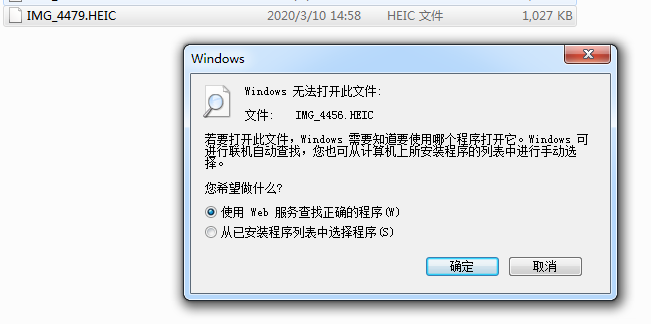heic图片是无法使用windows图片产查看器或者其他图片查看工具打开，因为ISO系统在更新到ios11版本后，iphone手机拍摄的文件格式为HEIC格式图片， 这种格式占用体积小，对于非苹果用户很不友好，所以在win7和win10系统下需要使用 “HEIC图片转换精灵” 将heic图片转成普通图片，将heic转成无损jpg,png,bmp 等常见格式,解决不同系统无法打开heic图片的限制. 支持系统： win7/win10 软件获取地址:www.heicghost.com 1.第一步打开安装的软件，设置输出格式，点击下拉列表选取转换后的格式，默认jpg,支持输出jpg,png,bmp ,然后点击添加文件夹按钮，批量导入文件，导入成功后文件列表显示导入的文件.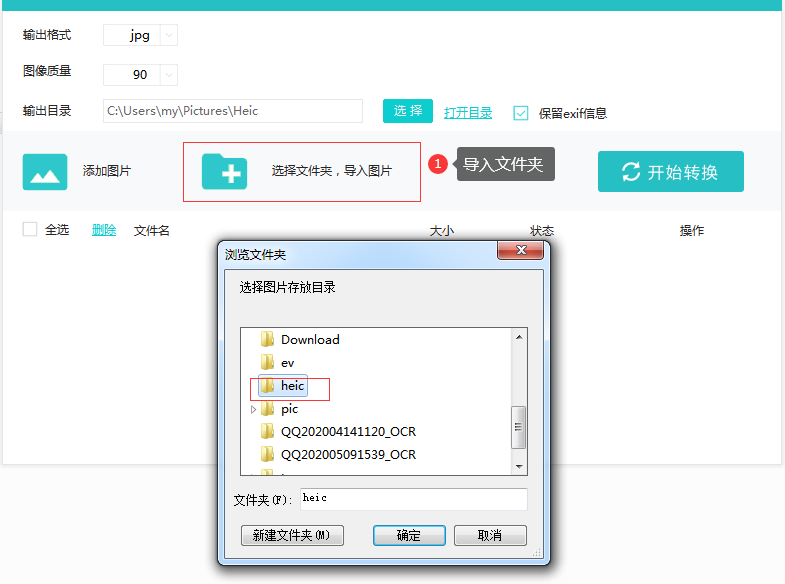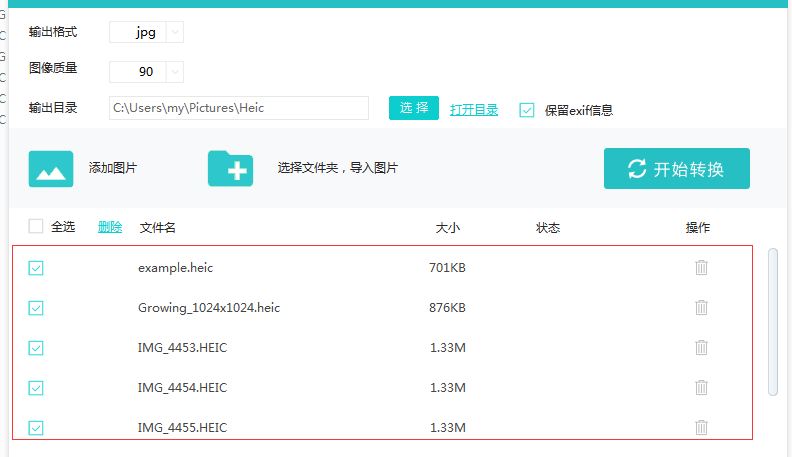2.第二步，列表中添加的文件默认是勾选的，只需一键点击开始转换，等待数秒钟，列表文件状态栏显示绿色的勾，代表文件转换完成，点击开目录，转换后jpg/pmg/bmp图片就在打开的目录下.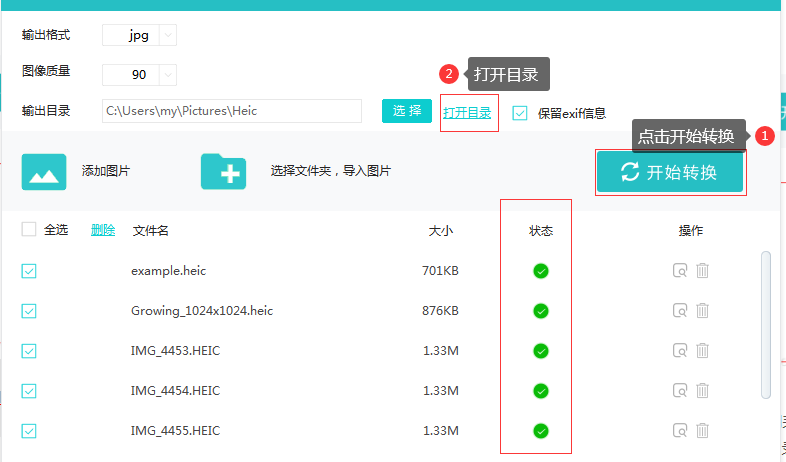展开全文heic转jpg heic转png • @ApiImplicitParam(name="receive_tel",value="收货人手机",paramType="query",required=false), @ApiImplicitParam(name="receive_provice",value="省",paramType="query",required=false), @...eclipse java • 我们多少都会接触到文件的使用情况，例如从手机的图库去取图片和视频，但是用intent调用，一般直接回返回来的一长串的地址，我们刚好需要的是文件名+后缀，等一些形式，这样子，就有时候我们就不知道怎么下手了，...  平常时，我们多少都会接触到文件的使用情况，例如从手机的图库去取图片和视频，但是用intent调用，一般直接回返回来的一长串的地址，我们刚好需要的是文件名+后缀，等一些形式，这样子，就有时候我们就不知道怎么下手了，但是有的人也会说，用正则表达式也可以啊，的确可以，但是我想我自己找网上找的别人的一些，不错的方法，也值得我们学习。也是我自己实际项目中用到的，就记录一下，方便自己学习。 上码:  public class GetFileName { public String getFileName(String pathandname) { /** * 仅保留文件名不保留后缀 */ int start = pathandname.lastIndexOf("/"); int end = pathandname.lastIndexOf("."); if (start != -1 && end != -1) { return pathandname.substring(start + 1, end); } else { return null; } } /** * 保留文件名及后缀 */ public String getFileNameWithSuffix(String pathandname) { int start = pathandname.lastIndexOf("/"); if (start != -1) { return pathandname.substring(start + 1); } else { return null; } } }  我们看看实际效果：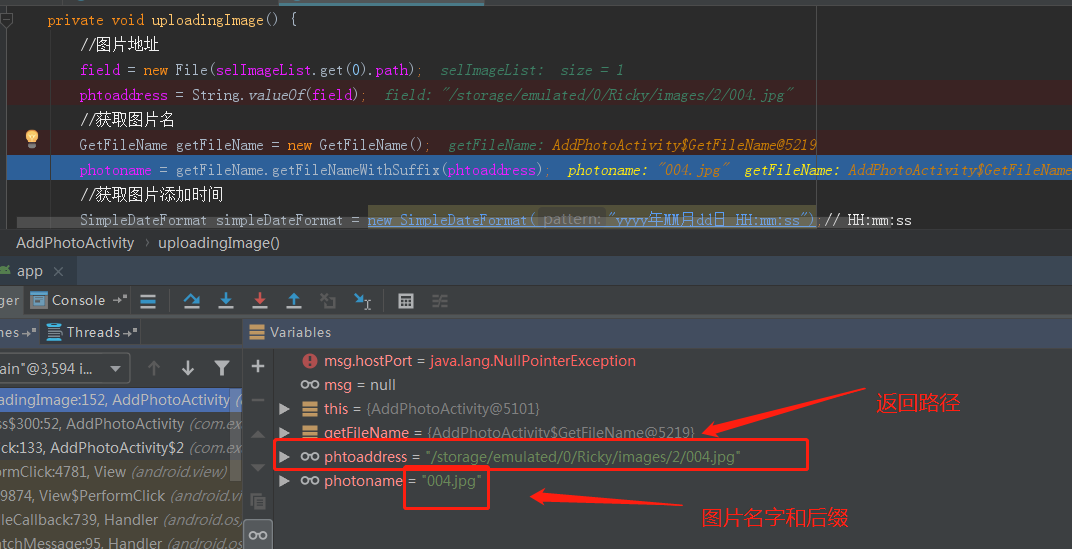其他文件名也可以参考这样去获取自己想要的文件名，前提是你先要拿到这文件的路径，你才可以去调用这个方法去实现。由于知识有限，有不足的，可以在【评论区】留意，我们一起去探讨学习，谢谢。  //获取本地文件夹的图片格式 private void filefload() { File scanner5Directory = new File(XXXXXXXXXX); if (scanner5Directory.isDirectory()) { for (File file : scanner5Directory.listFiles()) { String path = file.getAbsolutePath(); if (path.endsWith(".jpg") || path.endsWith(".png") || path.endsWith(".jpeg")) { list.add(path); } } } }   展开全文java • 那么图片肯定不能太大，但是目前手机端随便拍个照片都得个5M以上了，直接传上去的话那就太耗费线上资源了。怎么能把图片占用空间变小并且又能不太失真呢，那就用canvas试试！ 思路： 1、从设备选择图片 2、读取... 最近项目太太太紧张，一个月才憋出了这篇自认为有点干货的文章，先拿出来晒一晒，本文所有代码地址都可以到github下载或者直接npm install canvas-compression下载。 接下来 直奔主题： 背景：去年开发一个IM即时聊天系统，需要发送图片的功能。那么图片肯定不能太大，但是目前手机端随便拍个照片都得个5M以上了，直接传上去的话那就太耗费线上资源了。怎么能把图片占用空间变小并且又能不太失真呢，那就用canvas试试！ 思路： 1、从设备选择图片 2、读取文件 。2.1、转换file文件为base64 。2.2、获取文件拍摄方向信息 3、利用canvas压缩处理 。3.1、将图片绘制到canvas画布上 。3.2、根据设备信息判断绘制方式和是否旋转 4、上传前将文件从base64转换为file，并添加字段信息 5、上传至服务器 代码： 1、首先需要一个文件域  <div> <input type="file" accept="image/*" id="image"> </div> <p>压缩后的图片</p> <div id="compress"></div> <p>选择的原图片</p> <div id="proper"></div>  2、定义全局需要的变量  var active = function(){ this.image = document.getElementById('image') this.imgOrientation = null; // 设备方向 this.options={ field:'file',// 上传文件的字段 data:{'key': 'value'}, // 上传接口需要的附加参数 headers: {}, // 上传接口的请求头信息 width: 0, // 压缩后的图片宽度 当为0时会用原图片的宽度 quality: 0.6, // 压缩后图片的质量与原图的比例 }; };  3、选择图片后读取图片信息  // 拿到选择的图片 preview: function(){ var _this = this; this.image.addEventListener('change',function(val){ if(!val.currentTarget.files.length) return; // 单张上传，若需要多张上传此处需要做遍历 _this.createRender(val.currentTarget.files) }) }, // 拿到图片后，读取文件并获取图片拍摄方向 createRender: function(file){ var _this = this; var URL=window.URL || window.webkitURL, blob = URL.createObjectURL(file); if(window.FileReader){ var reader = new FileReader(); reader.readAsDataURL(file); reader.onload = function(){ var imgFalse = document.createElement('img'); // imgFalse.setAttribute('style','display:none;'); imgFalse.setAttribute('src',this.result); document.getElementById('proper').appendChild(imgFalse); imgFalse.onload=function(){ _this.imgOrientation=_this.getPhotoOrientation(imgFalse); _this.compress(blob , file); } } } },  4、利用canvas压缩处理 // 压缩操作（核心代码） compress: function(blob,file){ var _this = this; var img = new Image(); img.src = blob; img.onload = function() { var that = this; //生成比例 var w = that.width, h = that.height, scale = w / h; w = _this.options.width || w; h = w / scale; //生成canvas var canvas = document.createElement('canvas'); var ctx = canvas.getContext('2d'); canvas.setAttribute('width',w) canvas.setAttribute('height',h) ctx.drawImage(that, 0, 0, w, h); /** * 生成base64 */ var base64 = canvas.toDataURL('image/jpeg', _this.options.quality || 0.8); // 修复IOS if (navigator.userAgent.match(/iphone/i)) { // 宽高互换 canvas.setAttribute('width',h) canvas.setAttribute('height',w) ctx.translate(canvas.width / 2, canvas.height / 2); if(_this.imgOrientation=='6'){ //设置canvas宽高旋转90° ctx.rotate(90 * Math.PI / 180); }else if(_this.imgOrientation=='3'){ //设置canvas宽高旋转180° ctx.rotate(180 * Math.PI / 180); }else if(_this.imgOrientation=='8'){ //设置canvas宽高旋转270° ctx.rotate(270 * Math.PI / 180); } ctx.drawImage(that, 0, 0, w, h); ctx.drawImage(that, -this.width / 2, -this.height / 2); base64 = canvas.toDataURL('image/jpeg', _this.options.quality || 0.8); } // // 修复android if (navigator.userAgent.match(/Android/i)) { function JPEGEncoder(a){function I(a){var c,i,j,k,l,m,n,o,p,b=[16,11,10,16,24,40,51,61,12,12,14,19,26,58,60,55,14,13,16,24,40,57,69,56,14,17,22,29,51,87,80,62,18,22,37,56,68,109,103,77,24,35,55,64,81,104,113,92,49,64,78,87,103,121,120,101,72,92,95,98,112,100,103,99];for(c=0;64>c;c++)i=d((b[c]*a+50)/100),1>i?i=1:i>255&&(i=255),e[z[c]]=i;for(j=[17,18,24,47,99,99,99,99,18,21,26,66,99,99,99,99,24,26,56,99,99,99,99,99,47,66,99,99,99,99,99,99,99,99,99,99,99,99,99,99,99,99,99,99,99,99,99,99,99,99,99,99,99,99,99,99,99,99,99,99,99,99,99,99],k=0;64>k;k++)l=d((j[k]*a+50)/100),1>l?l=1:l>255&&(l=255),f[z[k]]=l;for(m=[1,1.387039845,1.306562965,1.175875602,1,.785694958,.5411961,.275899379],n=0,o=0;8>o;o++)for(p=0;8>p;p++)g[n]=1/(8*e[z[n]]*m[o]*m[p]),h[n]=1/(8*f[z[n]]*m[o]*m[p]),n++}function J(a,b){var f,g,c=0,d=0,e=new Array;for(f=1;16>=f;f++){for(g=1;g<=a[f];g++)e[b[d]]=[],e[b[d]]=c,e[b[d]]=f,d++,c++;c*=2}return e}function K(){i=J(A,B),j=J(E,F),k=J(C,D),l=J(G,H)}function L(){var c,d,e,a=1,b=2;for(c=1;15>=c;c++){for(d=a;b>d;d++)n[32767+d]=c,m[32767+d]=[],m[32767+d]=c,m[32767+d]=d;for(e=-(b-1);-a>=e;e++)n[32767+e]=c,m[32767+e]=[],m[32767+e]=c,m[32767+e]=b-1+e;a<<=1,b<<=1}}function M(){for(var a=0;256>a;a++)x[a]=19595*a,x[a+256>>0]=38470*a,x[a+512>>0]=7471*a+32768,x[a+768>>0]=-11059*a,x[a+1024>>0]=-21709*a,x[a+1280>>0]=32768*a+8421375,x[a+1536>>0]=-27439*a,x[a+1792>>0]=-5329*a}function N(a){for(var b=a,c=a-1;c>=0;)b&1<<c&&(r|=1<<s),c--,s--,0>s&&(255==r?(O(255),O(0)):O(r),s=7,r=0)}function O(a){q.push(w[a])}function P(a){O(255&a>>8),O(255&a)}function Q(a,b){var c,d,e,f,g,h,i,j,l,p,q,r,s,t,u,v,w,x,y,z,A,B,C,D,E,F,G,H,I,J,K,L,M,N,O,P,Q,R,S,T,U,V,W,X,Y,Z,$,_,k=0;const m=8,n=64;for(l=0;m>l;++l)c=a[k],d=a[k+1],e=a[k+2],f=a[k+3],g=a[k+4],h=a[k+5],i=a[k+6],j=a[k+7],p=c+j,q=c-j,r=d+i,s=d-i,t=e+h,u=e-h,v=f+g,w=f-g,x=p+v,y=p-v,z=r+t,A=r-t,a[k]=x+z,a[k+4]=x-z,B=.707106781*(A+y),a[k+2]=y+B,a[k+6]=y-B,x=w+u,z=u+s,A=s+q,C=.382683433*(x-A),D=.5411961*x+C,E=1.306562965*A+C,F=.707106781*z,G=q+F,H=q-F,a[k+5]=H+D,a[k+3]=H-D,a[k+1]=G+E,a[k+7]=G-E,k+=8;for(k=0,l=0;m>l;++l)c=a[k],d=a[k+8],e=a[k+16],f=a[k+24],g=a[k+32],h=a[k+40],i=a[k+48],j=a[k+56],I=c+j,J=c-j,K=d+i,L=d-i,M=e+h,N=e-h,O=f+g,P=f-g,Q=I+O,R=I-O,S=K+M,T=K-M,a[k]=Q+S,a[k+32]=Q-S,U=.707106781*(T+R),a[k+16]=R+U,a[k+48]=R-U,Q=P+N,S=N+L,T=L+J,V=.382683433*(Q-T),W=.5411961*Q+V,X=1.306562965*T+V,Y=.707106781*S,Z=J+Y,$=J-Y,a[k+40]=$+W,a[k+24]=$-W,a[k+8]=Z+X,a[k+56]=Z-X,k++;for(l=0;n>l;++l)_=a[l]*b[l],o[l]=_>0?0|_+.5:0|_-.5;return o}function R(){P(65504),P(16),O(74),O(70),O(73),O(70),O(0),O(1),O(1),O(0),P(1),P(1),O(0),O(0)}function S(a,b){P(65472),P(17),O(8),P(b),P(a),O(3),O(1),O(17),O(0),O(2),O(17),O(1),O(3),O(17),O(1)}function T(){var a,b;for(P(65499),P(132),O(0),a=0;64>a;a++)O(e[a]);for(O(1),b=0;64>b;b++)O(f[b])}function U(){var a,b,c,d,e,f,g,h;for(P(65476),P(418),O(0),a=0;16>a;a++)O(A[a+1]);for(b=0;11>=b;b++)O(B[b]);for(O(16),c=0;16>c;c++)O(C[c+1]);for(d=0;161>=d;d++)O(D[d]);for(O(1),e=0;16>e;e++)O(E[e+1]);for(f=0;11>=f;f++)O(F[f]);for(O(17),g=0;16>g;g++)O(G[g+1]);for(h=0;161>=h;h++)O(H[h])}function V(){P(65498),P(12),O(3),O(1),O(0),O(2),O(17),O(3),O(17),O(0),O(63),O(0)}function W(a,b,c,d,e){var h,l,o,q,r,s,t,u,v,w,f=e,g=e;const i=16,j=63,k=64;for(l=Q(a,b),o=0;k>o;++o)p[z[o]]=l[o];for(q=p-c,c=p,0==q?N(d):(h=32767+q,N(d[n[h]]),N(m[h])),r=63;r>0&&0==p[r];r--);if(0==r)return N(f),c;for(s=1;r>=s;){for(u=s;0==p[s]&&r>=s;++s);if(v=s-u,v>=i){for(t=v>>4,w=1;t>=w;++w)N(g);v=15&v}h=32767+p[s],N(e[(v<<4)+n[h]]),N(m[h]),s++}return r!=j&&N(f),c}function X(){var b,a=String.fromCharCode;for(b=0;256>b;b++)w[b]=a(b)}function Y(a){if(0>=a&&(a=1),a>100&&(a=100),y!=a){var b=0;b=50>a?Math.floor(5e3/a):Math.floor(200-2*a),I(b),y=a,console.log("Quality set to: "+a+"%")}}function Z(){var c,b=(new Date).getTime();a||(a=50),X(),K(),L(),M(),Y(a),c=(new Date).getTime()-b,console.log("Initialization "+c+"ms")}var d,e,f,g,h,i,j,k,l,m,n,o,p,q,r,s,t,u,v,w,x,y,z,A,B,C,D,E,F,G,H;Math.round,d=Math.floor,e=new Array(64),f=new Array(64),g=new Array(64),h=new Array(64),m=new Array(65535),n=new Array(65535),o=new Array(64),p=new Array(64),q=[],r=0,s=7,t=new Array(64),u=new Array(64),v=new Array(64),w=new Array(256),x=new Array(2048),z=[0,1,5,6,14,15,27,28,2,4,7,13,16,26,29,42,3,8,12,17,25,30,41,43,9,11,18,24,31,40,44,53,10,19,23,32,39,45,52,54,20,22,33,38,46,51,55,60,21,34,37,47,50,56,59,61,35,36,48,49,57,58,62,63],A=[0,0,1,5,1,1,1,1,1,1,0,0,0,0,0,0,0],B=[0,1,2,3,4,5,6,7,8,9,10,11],C=[0,0,2,1,3,3,2,4,3,5,5,4,4,0,0,1,125],D=[1,2,3,0,4,17,5,18,33,49,65,6,19,81,97,7,34,113,20,50,129,145,161,8,35,66,177,193,21,82,209,240,36,51,98,114,130,9,10,22,23,24,25,26,37,38,39,40,41,42,52,53,54,55,56,57,58,67,68,69,70,71,72,73,74,83,84,85,86,87,88,89,90,99,100,101,102,103,104,105,106,115,116,117,118,119,120,121,122,131,132,133,134,135,136,137,138,146,147,148,149,150,151,152,153,154,162,163,164,165,166,167,168,169,170,178,179,180,181,182,183,184,185,186,194,195,196,197,198,199,200,201,202,210,211,212,213,214,215,216,217,218,225,226,227,228,229,230,231,232,233,234,241,242,243,244,245,246,247,248,249,250],E=[0,0,3,1,1,1,1,1,1,1,1,1,0,0,0,0,0],F=[0,1,2,3,4,5,6,7,8,9,10,11],G=[0,0,2,1,2,4,4,3,4,7,5,4,4,0,1,2,119],H=[0,1,2,3,17,4,5,33,49,6,18,65,81,7,97,113,19,34,50,129,8,20,66,145,161,177,193,9,35,51,82,240,21,98,114,209,10,22,36,52,225,37,241,23,24,25,26,38,39,40,41,42,53,54,55,56,57,58,67,68,69,70,71,72,73,74,83,84,85,86,87,88,89,90,99,100,101,102,103,104,105,106,115,116,117,118,119,120,121,122,130,131,132,133,134,135,136,137,138,146,147,148,149,150,151,152,153,154,162,163,164,165,166,167,168,169,170,178,179,180,181,182,183,184,185,186,194,195,196,197,198,199,200,201,202,210,211,212,213,214,215,216,217,218,226,227,228,229,230,231,232,233,234,242,243,244,245,246,247,248,249,250],this.encode=function(a,b){var d,e,f,m,n,o,p,y,z,A,B,C,D,E,F,G,H,I,J,K,c=(new Date).getTime();for(b&&Y(b),q=new Array,r=0,s=7,P(65496),R(),T(),S(a.width,a.height),U(),V(),d=0,e=0,f=0,r=0,s=7,this.encode.displayName="_encode_",m=a.data,n=a.width,o=a.height,p=4*n,z=0;o>z;){for(y=0;p>y;){for(D=p*z+y,E=D,F=-1,G=0,H=0;64>H;H++)G=H>>3,F=4*(7&H),E=D+G*p+F,z+G>=o&&(E-=p*(z+1+G-o)),y+F>=p&&(E-=y+F-p+4),A=m[E++],B=m[E++],C=m[E++],t[H]=(x[A]+x[B+256>>0]+x[C+512>>0]>>16)-128,u[H]=(x[A+768>>0]+x[B+1024>>0]+x[C+1280>>0]>>16)-128,v[H]=(x[A+1280>>0]+x[B+1536>>0]+x[C+1792>>0]>>16)-128;d=W(t,g,d,i,k),e=W(u,h,e,j,l),f=W(v,h,f,j,l),y+=32}z+=8}return s>=0&&(I=[],I=s+1,I=(1<<s+1)-1,N(I)),P(65497),J="data:image/jpeg;base64,"+btoa(q.join("")),q=[],K=(new Date).getTime()-c,console.log("Encoding time: "+K+"ms"),J},Z()}function getImageDataFromImage(a){var d,b="string"==typeof a?document.getElementById(a):a,c=document.createElement("canvas");return c.width=b.width,c.height=b.height,d=c.getContext("2d"),d.drawImage(b,0,0),d.getImageData(0,0,c.width,c.height)} var encoder = new JPEGEncoder(); base64 = encoder.encode(ctx.getImageData(0, 0, w, h), _this.options.quality * 100 || 80); } // 生成结果 var result = { base64: base64, name:file.name, clearBase64: base64.substr(base64.indexOf(',') + 1) }; // 执行后函数 _this.beforeUpload(result,file); }; },  5、上传前将文件从base64转换为file，并添加字段信息上传回显  // 上传前处理数据 beforeUpload(result , file) { var formData = new FormData(); if(this.isIE()){ formData.append(this.options.field, file); }else{ formData.append(this.options.field, this.base64UrlToFile(result.base64,file.name)); } //追加额外的参数 var optionsData = this.options.data for(var x in optionsData){ optionsData[x] = typeof optionsData[x] === 'function' ? optionsData[x]():optionsData[x]; formData.append(x, optionsData[x]); } this.upload(formData ,result) }, // 上传操作 upload(formData,result){ // 实际应用中需要放开这段代码并引入jq //$.ajax({
//   url: ''
//   ,type: 'post'
//   ,data: formData
//   ,contentType: false
//   ,processData: false
//   ,dataType: 'text'
//   ,headers: _this.options.headers || {}
//   ,success: function(res){
// 成功后回显图片
var successImage = document.createElement('img');
// successImage.setAttribute('style','display:none;');
successImage.setAttribute('src',result.base64);
document.getElementById('compress').appendChild(successImage);
//   }
//   ,error: function(err){
//     console.log(err)
//     alert('请求失败')
//   }
// });
},

附：判断设备方向和文件转换等方法
	// 获取照片拍摄方向 需要引入EXIF.js
getPhotoOrientation: function(img){
var orient;
EXIF.getData(img, function () {
orient = EXIF.getTag(this, 'Orientation');
});
return orient;
},
// 判断是否ie浏览器
isIE: function() {
if(!!window.ActiveXObject || "ActiveXObject" in window){
return true;
}else{
return false;
}
},
//将base64转换为文件
base64UrlToFile: function (dataurl, filename) {
var arr = dataurl.split(','), mime = arr.match(/:(.*?);/),
bstr = atob(arr), n = bstr.length, u8arr = new Uint8Array(n);
while(n--){
u8arr[n] = bstr.charCodeAt(n);
}
return new File([u8arr], filename, {type:mime});
}

到此结束，搞定收工！


展开全文canvas html5 js
• 目前想通过控制APP扫码，然后选取相册的图片，但是appium获取不到图库里的元素，有没有高手知道怎么操作？？？ 2020年10月15日 1、争上游答题，只能虐菜了，50星以上基本上就干不过别人了。目前修了一稿，可以快速...
• 写一个接口根据手机获取会员所有收货地址列表，怎么做啊。我是刚刚毕业的学生，还不太会，求大神教我啊![图片说明](https://img-ask.csdn.net/upload/201611/21/1479707389_73129.png)asp.net
• iOS中启动屏后再加个闪屏 这个闪屏图片地址由后端获取，同时还有个MD5值（如果手机端网速太慢，可以先下载下来，在第二次启动app时再显示），这样怎么做好呢 求思路 就像网易新闻那样，启动页后面有个闪屏 但又不会...
• 1、首先在自己的网站上获取到企业微信登录的二维码（二维码怎么获取稍后再说，这里想象下你已经有了一个二维码） 2、你拿着手机扫描二维码，确认登录之后，页面就会自己进行重定向的跳转，跳转的url如何配置下面再...java spring http
• 微信二维码在微信的客户端...选择上传已经保存的二维码图片获取二维码的具体内容4.复制原始数据的地址链接，在二维码的文本里，重新生成二维码5、通过二维码美化功能，把二维码改写成你喜欢的样式，加上自己的商标。网络软件
• 3.选择上传已经保存的二维码图片获取二维码的具体内容 4.复制原始数据的地址链接，在二维码的文本里，重新生成二维码 5、通过二维码美化功能，把二维码改写成你喜欢的样式，加上自己的商标。
• xxx参数怎么获取？ 答：文档和博客均描述的很清楚，请仔细阅读。 我要xxxx，需要怎么做？ 答：看文档，看源码 网页每日更新的方式怎么做的？ 答：不是万能key。方案很简单，就是模拟点击+代理软件(Fiddler或...
• 　首页需要的数据是热门商品的信息，商品信息需要建立一个Bean对象，存储内容是商品的id、商品名称、商品单价、商品类别、商品数量、商品详细信息、商品成色、商品附带的图片地址、热门程度、发表用户的id、留言的...
• 重要：因为android 10 不支持用path路径显示图片，所以回调取消了单独获取path集合的回调，只保留获取Photo集合的回调，如需使用path，可以在Photo对象中获取 重要：ImageEngine接口（因为android 10 不支持用path...
• android看得到图片，ios手机看不到图片 这是wxparse解析器的问题 很多人都中枪~ 全局搜索并注释掉 console.dir 就好了 更多问题？ 如何升级到最新版 小程序程序的修改和您后台的数据是独立的，所以不用担心您...
• //在这个函数里面通过执行命令获取logcat的输出信息（输出信息里面包含了jni的崩溃地址），然后上传这个崩溃信息。 //当我们获取到崩溃信息后，可以通过arm-linux-androideabi-addr2line（具体可能不是这个名字，在...
• XIU主题是一款清新扁平风格、13种颜色、多设备支持、SEO优化、各种提速优化的Wordpress主题. 功能展示 清新·扁平:前端展示小清新，扁平化设计风格 ...修复手机端相关文章图片问题。 演示地址：http://blog.weknow.cn
• ApiImpl Api接口 实现了地址查询 身份证查询 手机信息查询 更新信息查询 网络请求的功能 AddressInfo 定义了一个地址信息结构类 AppUpdateInfo定义了一个更新信息结构类 IDCardInfo 定义了一个身份证结构类 ...
• 可以对URL进行改名，URL地址怎么变就怎么变。 3.完美的 帐务管理 当时用户交易情况如 交易金额;交易对象;资金流向等详细记录,并与订单关联，可以追踪到某个任务上。 交易金额的来龙去脉一目了然。 4.完美的 ...
• 项目地址： 链接: https://pan.baidu.com/s/1OHSva0M5PIQMWNP0iwzlLg 提取码: csf5 求大能帮我解决一下。我不知道怎么去写这个跳转。 ![图片说明]...android xml
• 正如我在某次“还我旧版”运动中听到的声音，“不管怎么改版，只要友邻们还在就好”，改版是豆瓣不断良好发展的必经之路，但这句话中对友邻的珍重又令我感受到了豆瓣最宝贵的特质。 豆瓣作为一个工具的价值可以通过...
• 16.2.5 获取运行时信息 326 16.2.6 文件浏览器 328 16.2.7 项目的细节完善 331 16.3 手机信息查看助手功能展望 332 16.4 项目的打包、签名和发布 332 16.5 本章小结 333 第17章 芝麻开门——Android底层开发和...
• ColorMatrix处理图片↘颜色矩阵.js ColorMatrix颜色矩阵的用法.js common(1).js common.js config.js DailyTask.js DensityUtil.js didi.js digit.js dou_yin_yang_hao(1).js dou_yin_yang_hao.js ...
• ## vc++ 应用源码包_1

热门讨论 2012-09-15 14:22:12
内含（重启、图片操作、ip操作、键盘与鼠标、客户端以及服务端、文件传输等实例源码） 多个VC++加密解密算法库（CRYPT++） 详细讲解了Crypt++的加密解密的使用以及其它的加密解密方法（例如base64加解密、哈希加...
• ## vc++ 应用源码包_2

热门讨论 2012-09-15 14:27:40
内含（重启、图片操作、ip操作、键盘与鼠标、客户端以及服务端、文件传输等实例源码） 多个VC++加密解密算法库（CRYPT++） 详细讲解了Crypt++的加密解密的使用以及其它的加密解密方法（例如base64加解密、哈希加...
• ## vc++ 应用源码包_6

热门讨论 2012-09-15 14:59:46
内含（重启、图片操作、ip操作、键盘与鼠标、客户端以及服务端、文件传输等实例源码） 多个VC++加密解密算法库（CRYPT++） 详细讲解了Crypt++的加密解密的使用以及其它的加密解密方法（例如base64加解密、哈希加...
• ## vc++ 应用源码包_5

热门讨论 2012-09-15 14:45:16
内含（重启、图片操作、ip操作、键盘与鼠标、客户端以及服务端、文件传输等实例源码） 多个VC++加密解密算法库（CRYPT++） 详细讲解了Crypt++的加密解密的使用以及其它的加密解密方法（例如base64加解密、哈希加...